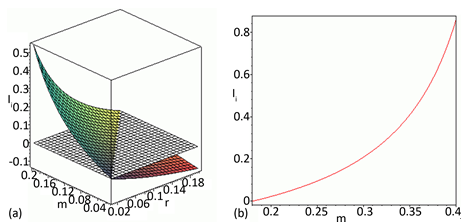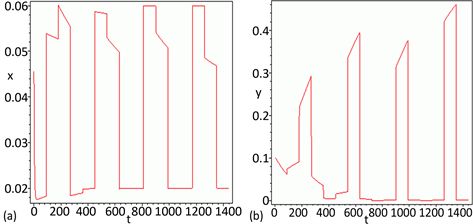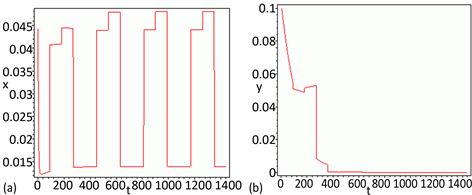﻿ 一类水体富营养化复杂动力系统构建及其动力学分析

# 一类水体富营养化复杂动力系统构建及其动力学分析Dynamics Analysis and Construction of a Water Eutrophication Complex Dynamical System

Abstract: In this paper, firstly, on the basis of research progress of water eutrophication in Wujiayuan res-ervoir, a distributed function is introduced to characterize the total phosphorus input being changed with the season in the process of dynamic modeling, a water eutrophication complex dy-namical system has been structured. Secondly, some theoretical analysis and numerical simulation on the complex dynamical system have been investigated to establish some judgment criterions for the asymptotic stability of the internal equilibrium point and describe the dynamic trends of total phosphorus concentration and algal population density, which can dissect the interaction mechanism between total phosphorus and algal population, and then reveal the influence mecha-nism of total phosphorus input control strategy on eutrophication status and algal bloom. Finally, these studies can provide certain theoretical basis for the further prediction of the nutrient dy-namic evolution trend and the deep exploration of the algae population growth dynamic law in Wujiayuan reservoir.

1. 引言

2. 动态建模

$\left\{\begin{array}{l}\frac{\text{d}x\left(t\right)}{\text{d}t}={I}_{i}-bx\left(t\right)-\frac{ax\left(t\right)y\left(t\right)}{c+x\left(t\right)}\\ \frac{\text{d}y\left(t\right)}{\text{d}t}=ry\left(t\right)+\frac{aex\left(t\right)y\left(t\right)}{c+x\left(t\right)}-my\left(t\right)\end{array}$ (1)

3. 理论分析

$\left\{\begin{array}{l}{I}_{i}-bx\left(t\right)-\frac{ax\left(t\right)y\left(t\right)}{c+x\left(t\right)}=0\\ r+\frac{aex\left(t\right)}{c+x\left(t\right)}-m=0\end{array}$ (2)

$u\left(t\right)=x\left(t\right)-{x}_{1},\text{ }\text{ }\text{ }\text{ }\text{ }\text{ }\text{ }\text{ }v\left(t\right)=y\left(t\right)-{y}_{1}$ (3)

$\left\{\begin{array}{l}\frac{\text{d}u\left(t\right)}{\text{d}t}={a}_{11}u\left(t\right)+{a}_{12}v\left(t\right)\\ \frac{\text{d}v\left(t\right)}{\text{d}t}={a}_{21}u\left(t\right)+{a}_{22}v\left(t\right)\end{array}$ (4)

${a}_{11}=-b-\frac{{I}_{i}{\left(ae+r-m\right)}^{2}-bc\left(m-r\right)\left(ae+r-m\right)}{aec\left(m-r\right)}$ , ${a}_{12}=\frac{r-m}{e}$

${a}_{21}=\frac{{I}_{i}{\left(ae+r-m\right)}^{2}-bc\left(ae+r-m\right)\left(m-r\right)}{ac\left(m-r\right)}$ , ${a}_{22}=0$

4. 数值模拟与分析

5. 结论Figure 1. The value distribution trend of key parametersFigure 2. The simulated dynamic evolution trend of total phosphorus concentration and algal population density of the system (1)Figure 3. The simulated dynamic evolution trend of total phosphorus concentration and algal population density of the system (1) under the condition of controlled total phosphorus

 Ryding, S. and Rast, W. (1989) The Control of Eutrophication of Lakes and Reservoirs. The Parthenon Publishing Group, Nashville.

 Qin, B.Q. (2009) Lake Eutrophication: Control Countermeasures and Recycling. Ecological En-gineering, 35, 1569-1573.
https://doi.org/10.1016/j.ecoleng.2009.04.003

 高月香, 张永春. 水文气象因子对藻华爆发的影响[J]. 水科学与工程技术, 2006(2): 10-12.

 赵磊, 刘永, 李玉照, 等. 胡泊生态系统稳定转换理论和驱动因子研究进展[J]. 生态环境科学, 2014, 23(10): 1697-1707.

 刘玉生, 韩梅, 梁占彬, 林毅雄. 光照、温度和营养盐对滇池微囊藻生长的影响[J]. 环境科学研究, 1995, 8(6): 8-11.

 周慧敏, 冯剑丰, 朱琳, 李文娇. 温度、光照和磷酸盐脉冲输入对三角褐指藻的交互影响[J]. 中国环境科学, 2015, 35(1): 244-250.

 高学庆, 任久长, 宗志祥, 蔡晓明. 铜绿微囊藻营养动力学研究[J]. 北京大学学报(自然科学版), 1994, 30(4): 462-469.

 刘春光, 金相灿, 邱金泉, 等. 光照和磷的交互作用对两种淡水藻类生长的影响[J]. 中国环境科学, 2005, 25(1): 32-36.

 Mei, Z.P., Finkel, Z.V. and Irwin, A.J. (2009) Light and Nutrient Availability Affect the Size-Scaling of Growth in Phytoplankton. Journal of Theoretical Biology, 259, 582-588.
https://doi.org/10.1016/j.jtbi.2009.04.018

 Yang, M., Zhao, W. and Xie, X. (2014) Effects of Nitrogen, Phosphorus, Iron and Silicon on Growth of Five Species of Marine Benthic Diatoms. Acta Ecologica Sinica, 34, 311-319.
https://doi.org/10.1016/j.chnaes.2014.10.003

 Lv, J., Wu, H.J. and Chen, M.Q. (2011) Effects of Nitrogen and Phosphorus on Phytoplankton Composition and Biomass in Subtropical, Urban Shallow Lakes in Wuhan, China. Limnologica, 41, 48-56.
https://doi.org/10.1016/j.limno.2010.03.003

 Huisman, J. and Weissing, F.J. (1994) Light-Limited Growth and Competition for Light in Well-Mixed Aquatic Environments: An Elementary Model. Ecology, 75, 507-520.
https://doi.org/10.2307/1939554

 Huisman, J., Jonker, R.R., Zonneveld, C. and Weissing, F.J. (1999) Com-petition for Light between Phytoplankton Species: Experimental Tests of Mechanistic Theory. Ecology, 80, 211-222.
https://doi.org/10.1890/0012-9658(1999)080[0211:CFLBPS]2.0.CO;2

 Huisman, J., Pham Thi, N.N., Karl, D.M. and Sommeijer, B. (2006) Reduced Mixing Generates Oscillations and Chaos in the Oceanic Deep Chlorophyll Maximum. Nature, 439, 322-325.
https://doi.org/10.1038/nature04245

 Huisman, J. and Welssing, F.J. (1999) Biodiversity of Plankton by Species Oscillations and Chaos. Nature, 402, 407-410.
https://doi.org/10.1038/46540

 许秋瑾, 秦伯强, 陈伟民, 陈灿宇, 高光. 太湖藻类生长模型研究[J]. 胡泊科学, 2011, 13(2): 149-156.

 刘晓臣, 李小平, 王玉峰, 等. 基于生态动力学模型的兴凯湖营养物质入湖与富营养化状态响应模拟[J]. 湖泊科学, 2013, 25(6): 862-871.

 Chen, S.N., Chen, X.L., Peng, Y. and Peng, K.D. (2009) A Mathematical Model of the Effect of Nitrogen and Phosphorus on the Growth of Blue-Green Lagae Population. Applied Mathematical Modelling, 33, 1097-1106.
https://doi.org/10.1016/j.apm.2008.01.001

 Yu, H.G., Zhao, M., Wang, Q. and Agarwal, R.P. (2014) A Focus on Long-Run Sustainability of an Impulsive Switched Eutrophication Controlling System Based upon the Zeya Reservoir. Journal of the Franklin Institute, 351, 487-499.
https://doi.org/10.1016/j.jfranklin.2013.08.025

 Yu, H.G., Zhao, M. and Agarwal, R.P. (2014) Stability and Dynamics Analysis of Time Delayed Eutrophication Ecological Model Based upon the Zeya Reservoir. Mathematics and Computers in Simulation, 97, 53-67.
https://doi.org/10.1016/j.matcom.2013.06.008

 Steel, J.A. (1995) Modelling Adaptive Phytoplankton in a Variable Environment. Ecological Modelling, 78, 117-127.
https://doi.org/10.1016/0304-3800(94)00122-X

 Alpine, A.E. and Cloern, J.E. (1988) Phytoplankton Growth Rates in a Light-Limited Environment, San Francisco Bay. Marine Ecology-Progress Series, 44, 167-173.
https://doi.org/10.3354/meps044167

 Li, X.D. and Song, S. (2017) Stabilization of Delay Systems: De-lay-Dependent Impulsive Control. IEEE Transactions on Automatic Control, 62, 406-411.
https://doi.org/10.1109/TAC.2016.2530041

 Li, X.D., Zhang, X. and Song, S. (2017) Effect of Delayed Im-pulses on Input-to-State Stability of Nonlinear Systems. Automatica, 76, 378-382.
https://doi.org/10.1016/j.automatica.2016.08.009

 Li, X.D. and Cao, J. (2017) An Impulsive Delay Inequality Involving Unbounded Time-Varying Delay and Applications. IEEE Transactions on Automatic Control, 62, 3618-3625.
https://doi.org/10.1109/TAC.2017.2669580

 Li, X.D. and Song, S. (2013) Impulsive Control for Existence, Uniqueness and Global Stability of Periodic Solutions of Recurrent Neural Networks with Discrete and Continuously Distributed Delays. IEEE Transactions on Neural Networks and Learning Systems, 24, 868-877.
https://doi.org/10.1109/TNNLS.2012.2236352

 Xu, L.G. and He, D.H. (2011) Mean Square Exponential Stability Analysis of Impulsive Stochastic Switched Systems with Mixed Delays. Computers and Mathematics with Application, 62, 109-117.
https://doi.org/10.1016/j.camwa.2011.04.056

 Liu, X., Zhong, S.M. and Ding, X.Y. (2012) Robust Exponen-tial Stability of Impulsive Switched Systems with Switching Delays: A Razumikhin Approach. Communications in Nonlinear Science and Numerical Simulation, 17, 1805-1812.
https://doi.org/10.1016/j.cnsns.2011.09.013

 Lee, S.H. and Lim, J.T. (1999) Stability Analysis of Switched Systems with Impulse Effects. Proceedings of the 1999 IEEE, International Symposium on Intelligent Control intelligent Systems and Semiotics, Cambridge, MA, September 1999, 15-17.

 Liu, Y.Q., Eberhard, A. and Teo, K.L. (2006) A Numerical Method for a Class of Mixed Switching and Impulsive Optimal Control Problems. Computers & Mathematics with Applications, 52, 625-636.
https://doi.org/10.1016/j.camwa.2006.10.001

Top